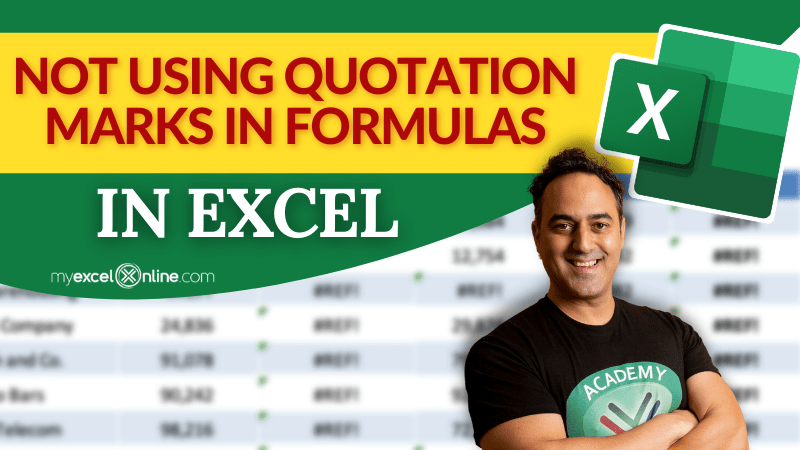Did you know? While working on a formula in Excel, the text should be written in quotes for Excel to recognize it as text.

Excel is by default programmed to accept cell references as the arguments for its formulas, and inserting texts without quotation marks will return an error.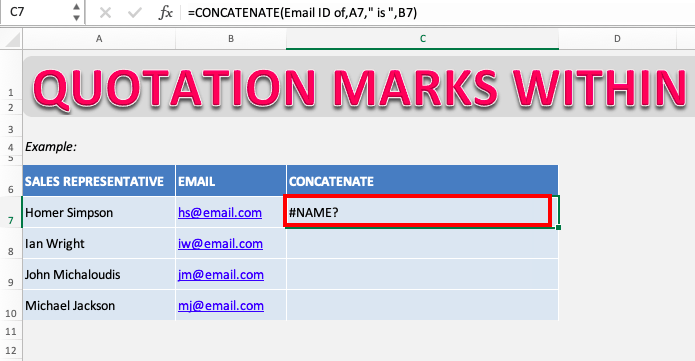Let us understand this issue better with an example!

Taking the concatenate formula, its primary function would be to join two or more text strings together.

#### =CONCATENATE(Email ID of,A7,” is “,B7)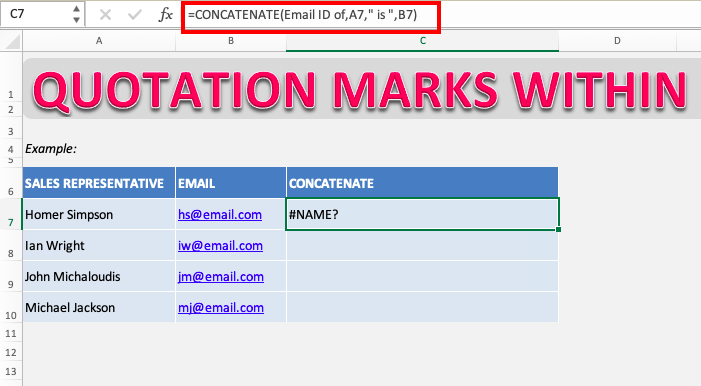As you can see, we have entered a couple of text strings as the concatenate formula’s argument, but Excel is returning the value as an error.

This is because the text strings (i.e. Email ID of) that we have entered are not in quotation marks, and hence Excel is unable to comprehend the values given as arguments.

Even though concatenate is a function largely used to combine text strings, Excel is unable to identify text unless:

Let’s try this formula again in the correct way!

### Using Cell References

Here we will use the same concatenate function by given cell references that contain the texts we want to join.

As you can see, the cells B6 and C6 contain the text we want to join. Simply selecting the cells as each of the arguments for the function will return the value it is!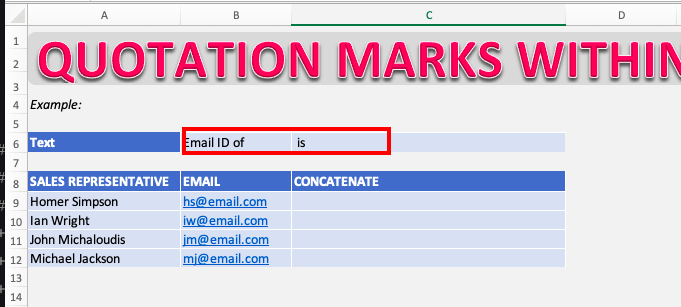You can use this formula to concatenate using cell reference:

#### =CONCATENATE(\$B\$6,A9,\$C\$6,B9)

Make sure to put \$ signs to freeze the cell reference so that you can easily copy the formula down!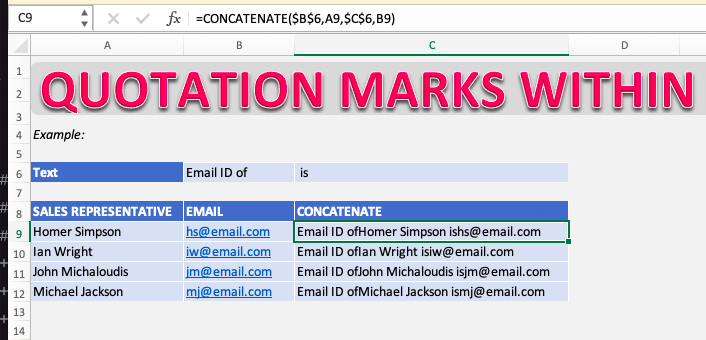This might create an issue as there is no space in between the texts.

So after each cell reference given in the arguments, we must insert a space. To do this, we just have to add ” ” in-between the text references to add a space in between.

And, Voila. You now have the desired result!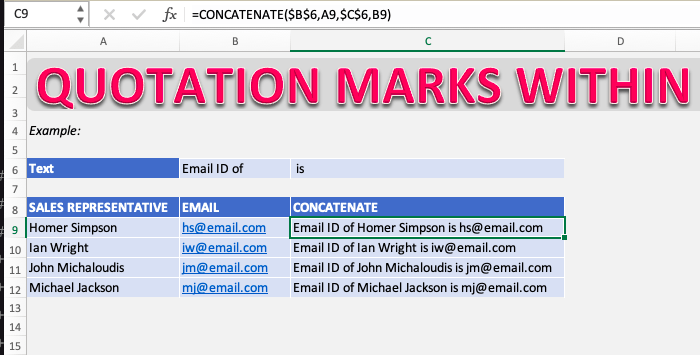### Using Quotation Marks

As explained above, text that is to be entered within the arguments of formula must be in quotation marks.

#### =CONCATENATE(“Email ID of “,A7,” is “,B7)

Make sure to add space right after the text!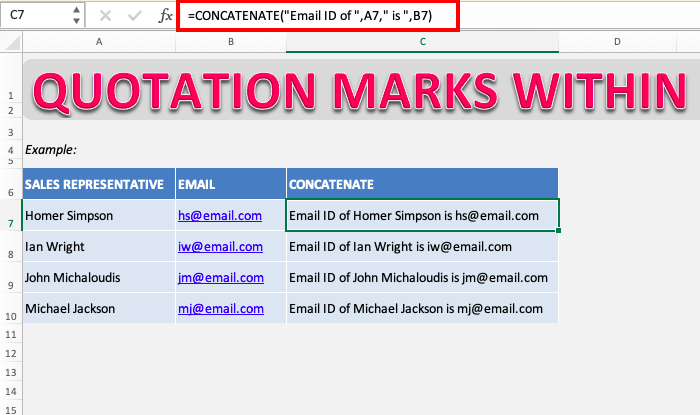Here we have entered a couple of texts as arguments in the concatenate function, and notice how every text is in quotation marks. These same text strings returned an error when we used them without quotation marks.

Similarly, here we can also add spaces in between in-text as explained above.

### Conclusion

When writing formulas, Excel will identify a text only if it’s written within quotation marks.

Excel extracts anything written within the quotation mark as text and discards the quotation mark. We hope after this exercise, the concept of inserting text within quotes is clear to you and you can now use Excel formulas efficiently.